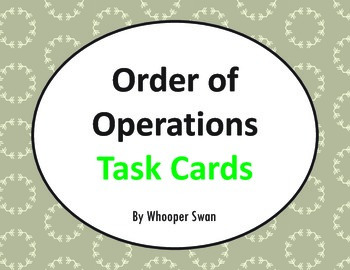## Order of Operations Task Cards\$2.00In this packet you will find 24 Task Cards, Recording Sheet and Answer key.

Print on cardstock, laminate and cut out each set of cards.

Cards 1-4) 3 numbers, addition, subtraction and parentheses.
Example: 3 + (2 - 1) =

Cards 5-8) 4 numbers, addition, subtraction and parentheses.
Example: 4 - 4 + (8 - 2) =

Cards 9-12) 3 numbers, addition, subtraction, multiplication and parentheses.
Example: (2 x 2) - 1 =

Cards 13-16) 4 numbers, addition, subtraction, multiplication and parentheses.
Example: 4 - (4 x 1 + 2) =

Cards 17-20) 3 numbers, addition, subtraction, multiplication, division and parentheses.
Example: (4 ÷ 2) + 3 =

Cards 21-24) 4 numbers, addition, subtraction, multiplication, division and parentheses.
Example: (9 ÷ 3 + 6) x 1 =

Contains negative numbers.
----------------------------------

You May Also Like These worksheets:
Order of Operations Worksheets

Be the first to know about my new discounts, freebies and product launches: